﻿ ﻿﻿﻿ Fractional Calculus Computer Algebra System math software Home | list | math | function | calculator | handbook | help | forum | index | 中文++=﻿

# Fractional Calculus 分数阶微积分

1. ## Input Fractional Calculus into Calculator

Input sin(0.5,x) for the 0.5th order derivative of sin(x), which is denoted by sin^((0.5))(x). It is differrent from power of sin(x) as sin(x)^2. sin(0.5,x) can be computed and plot. d(sin(x),x,0.5) computes the 0.5th order derivative of sin(x). If you want to hold it (not evaluate), use the derivative holder ds(y,x,0.5).

2. ## Definition and property of Fractional Calculus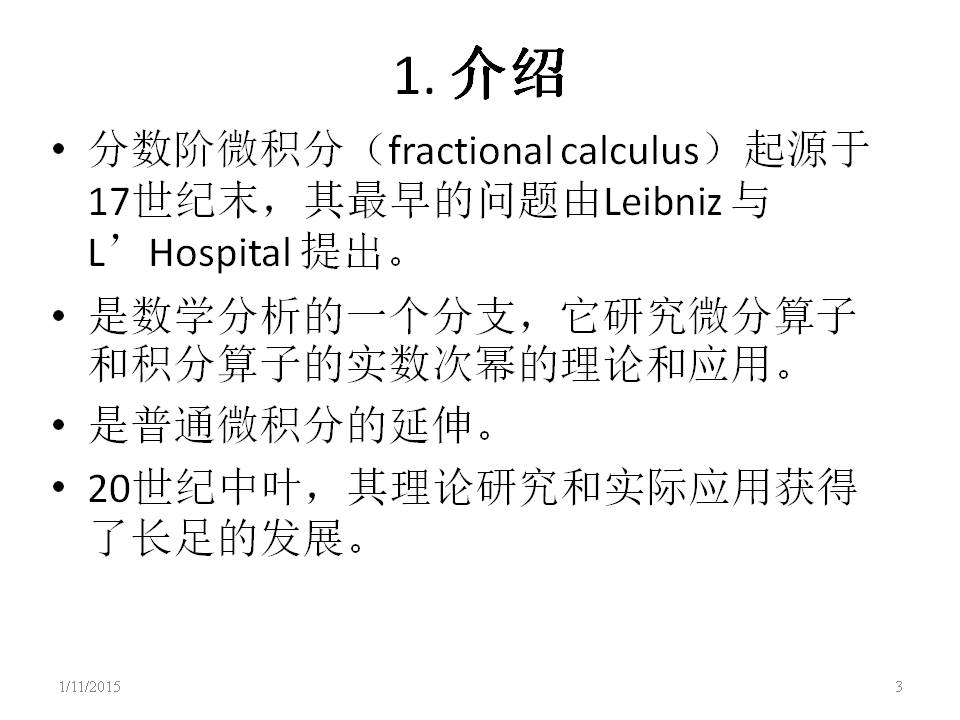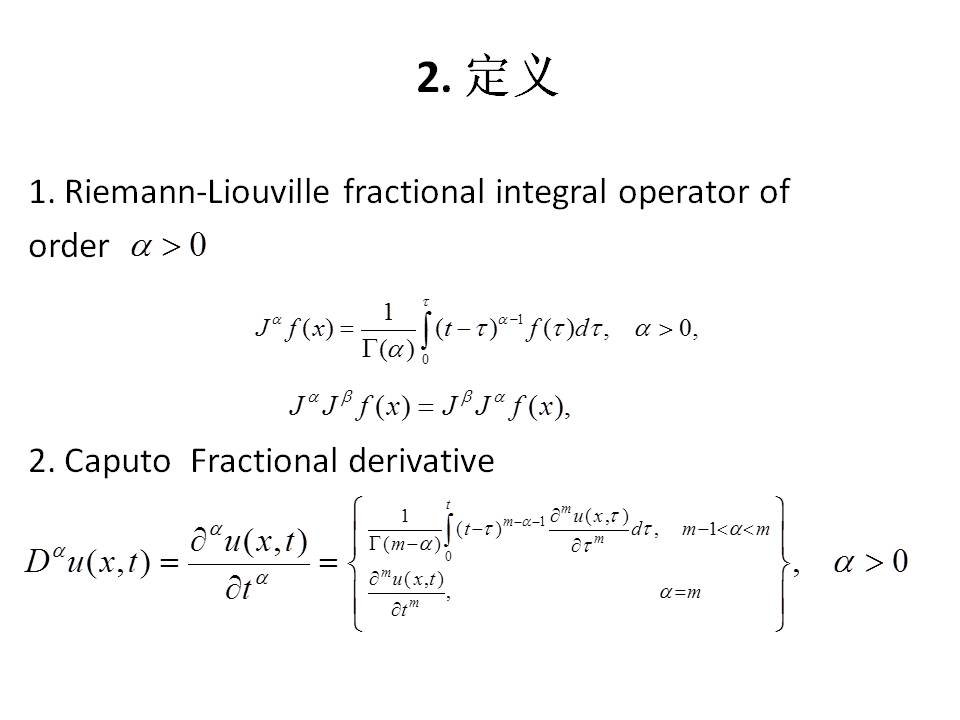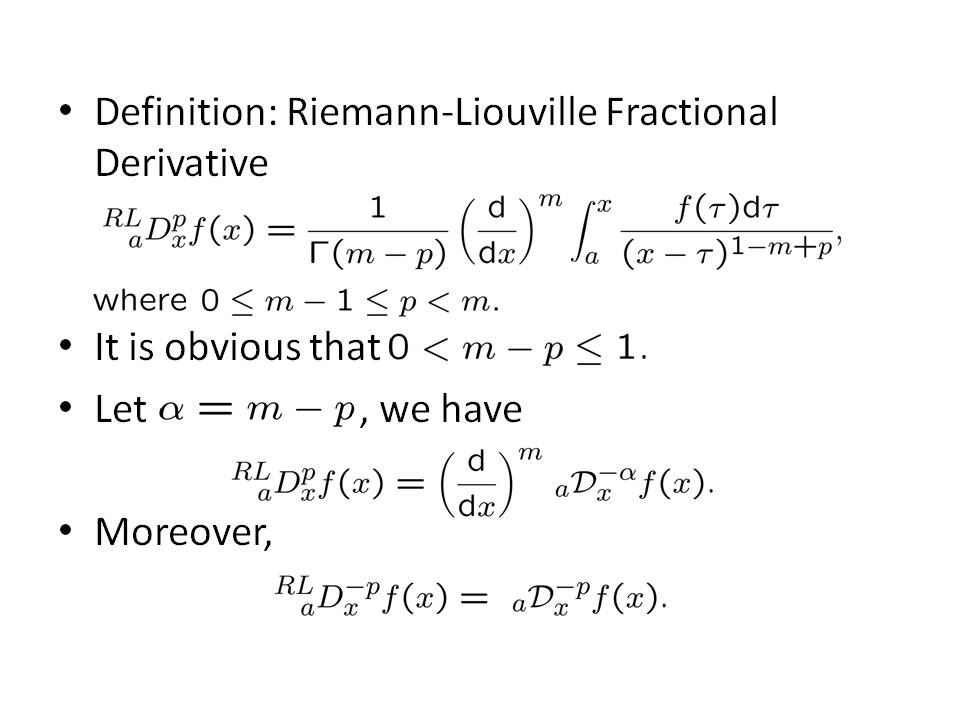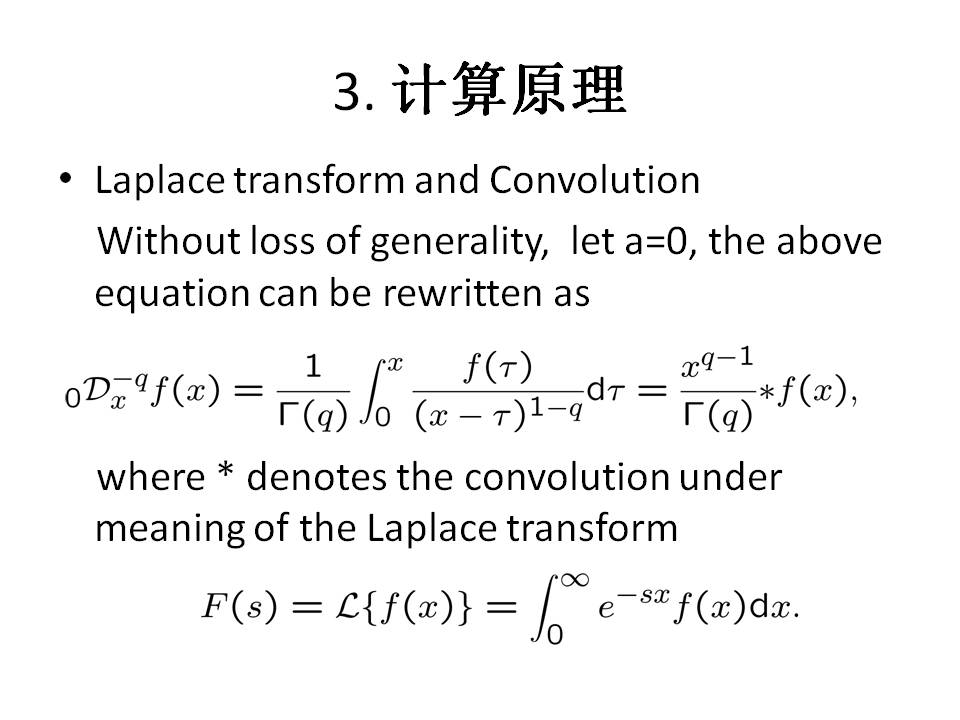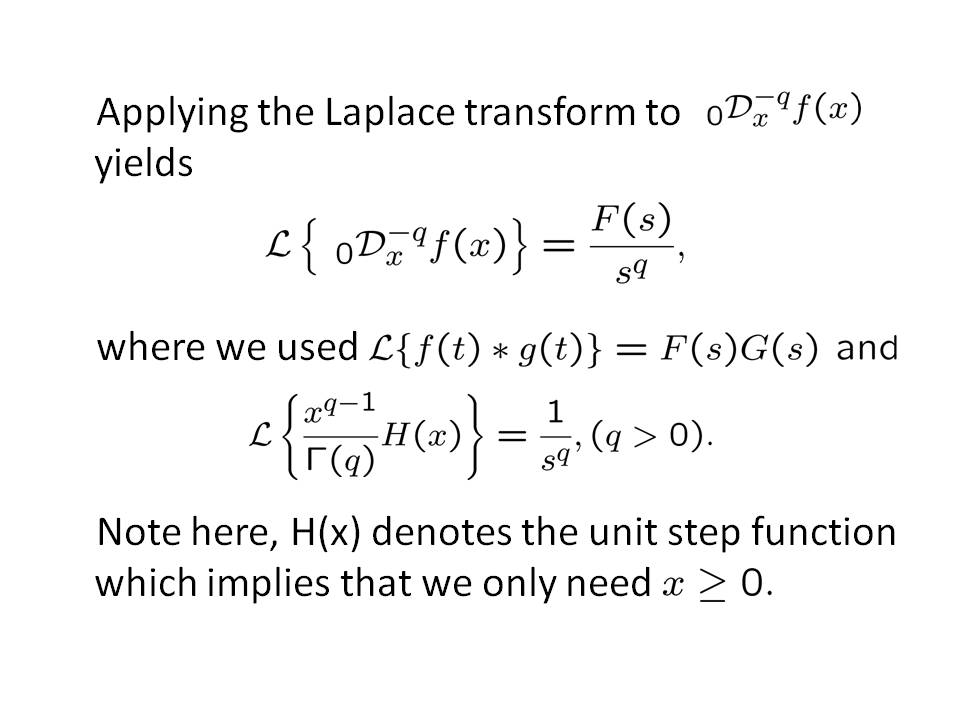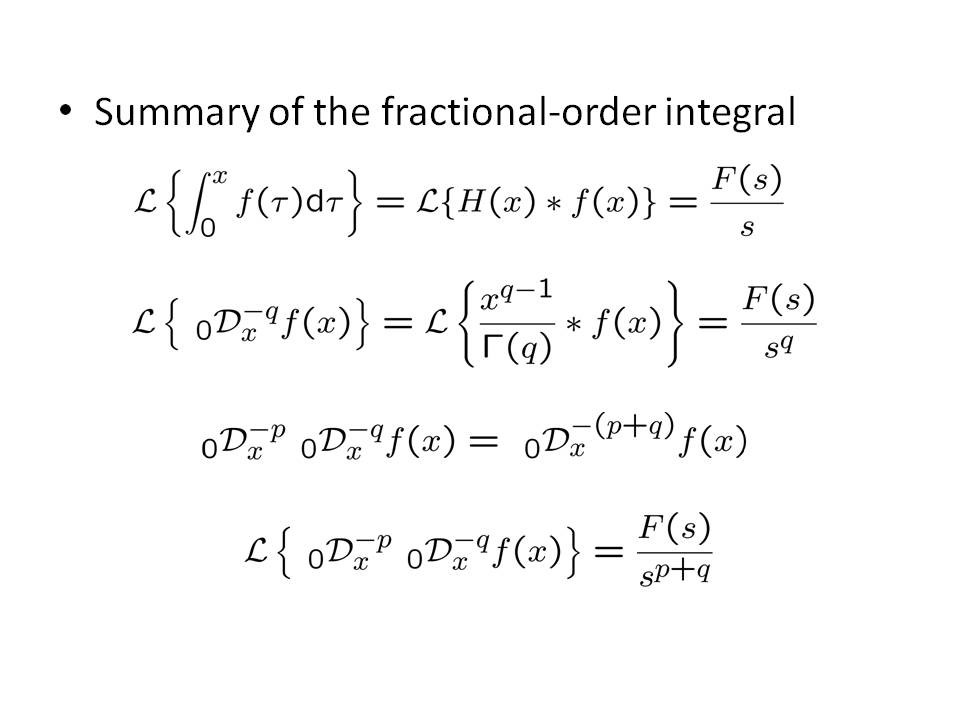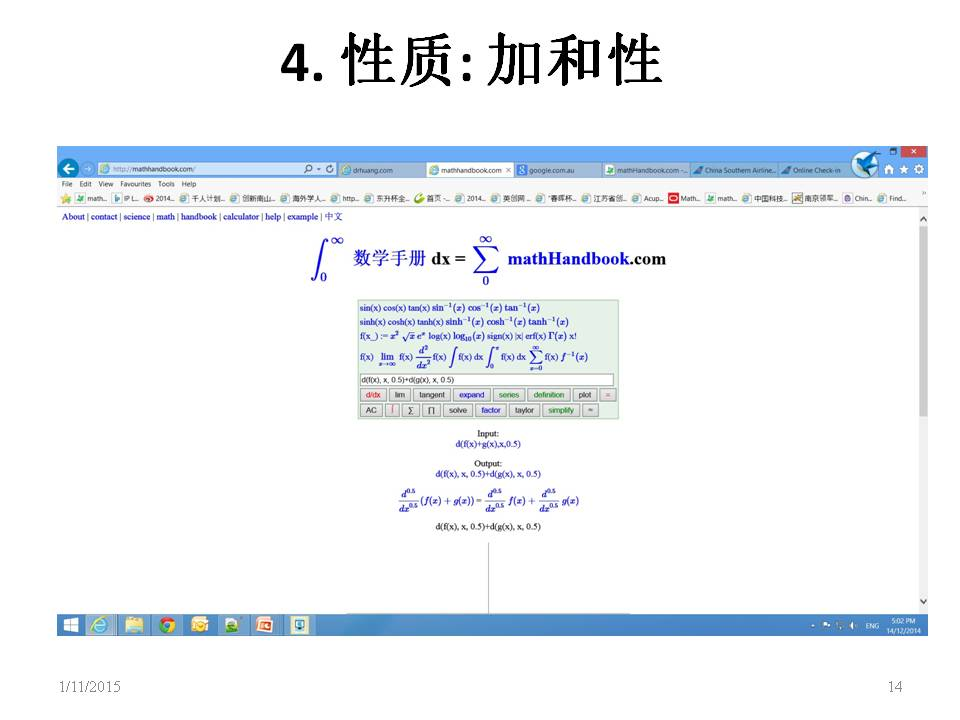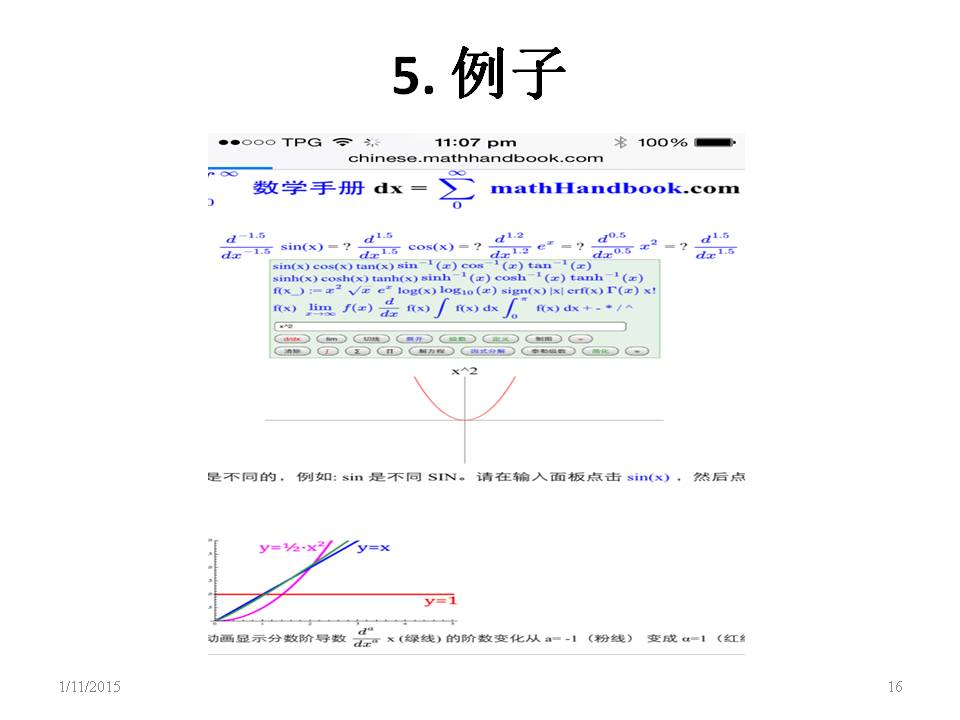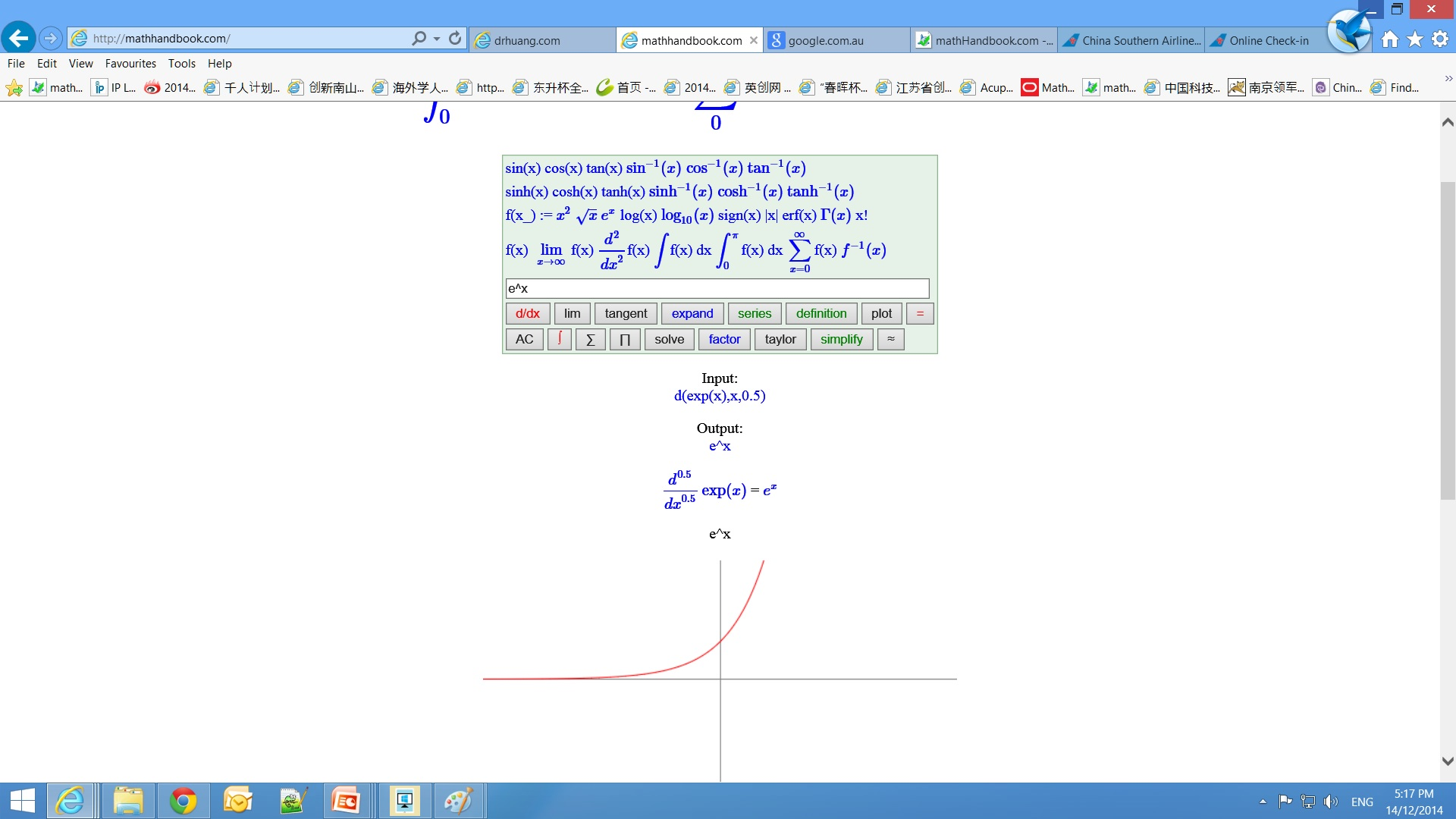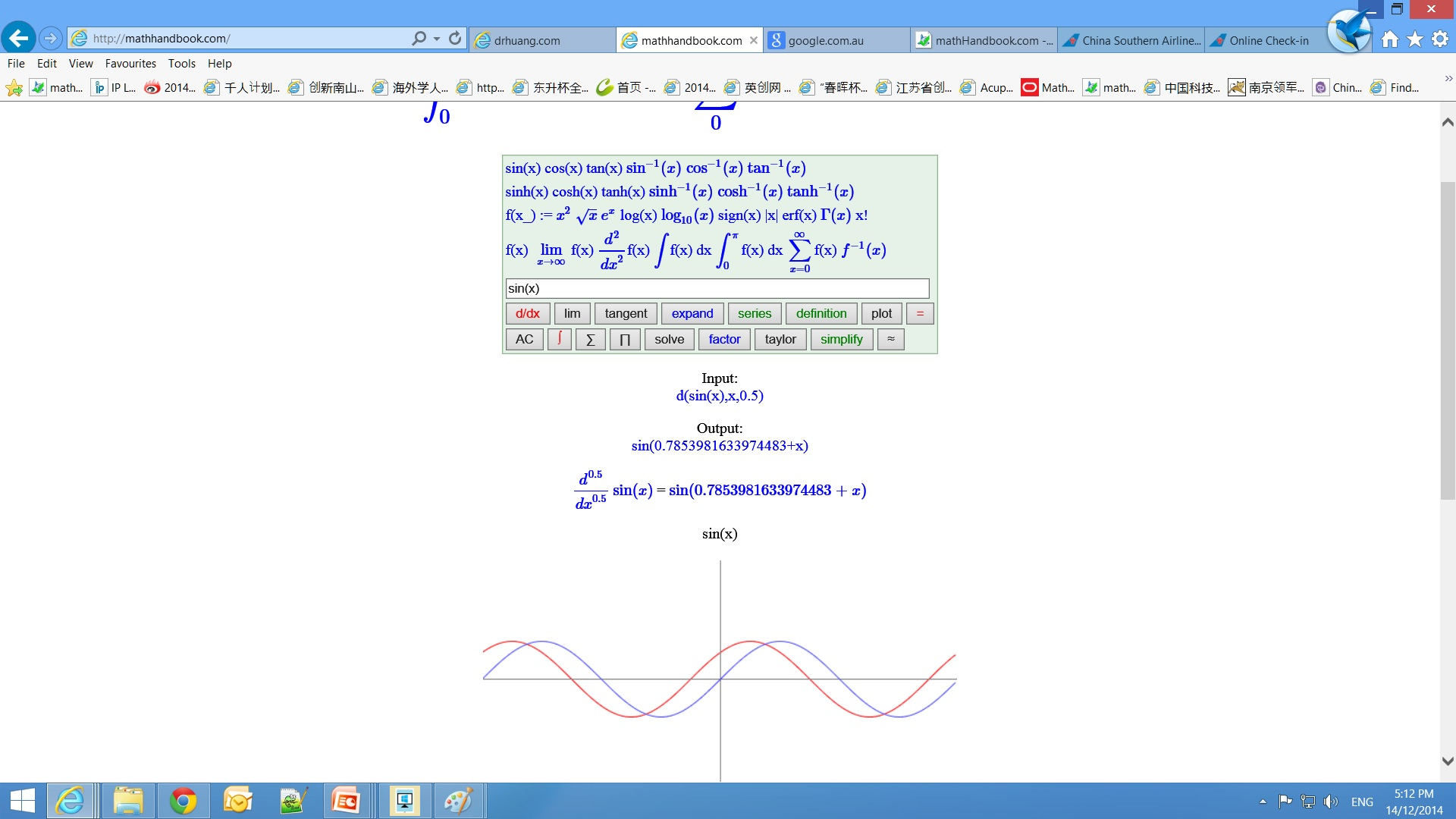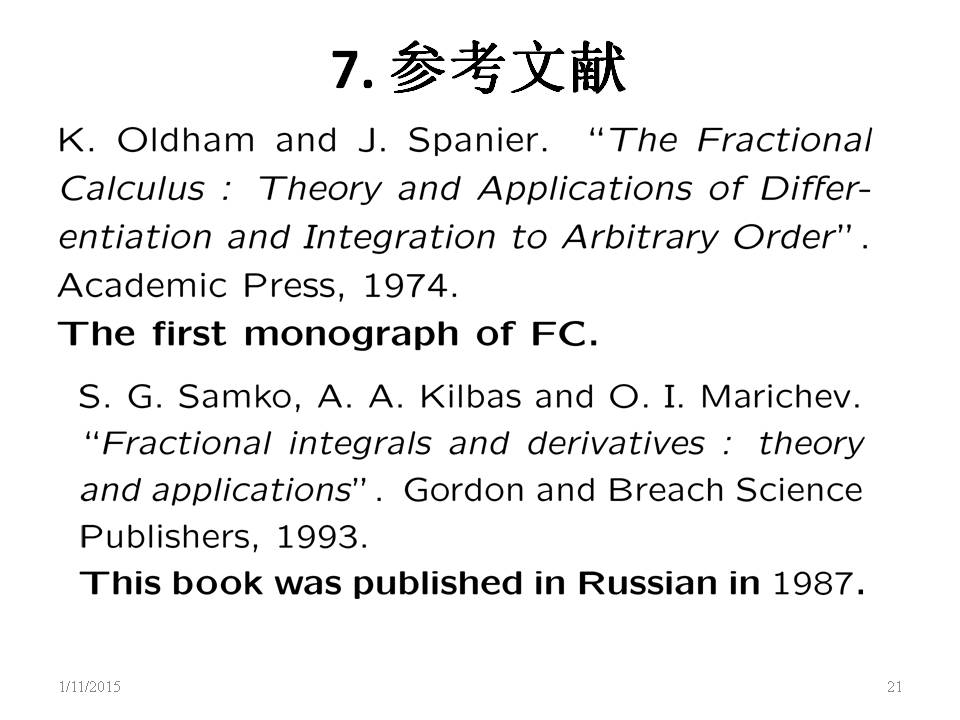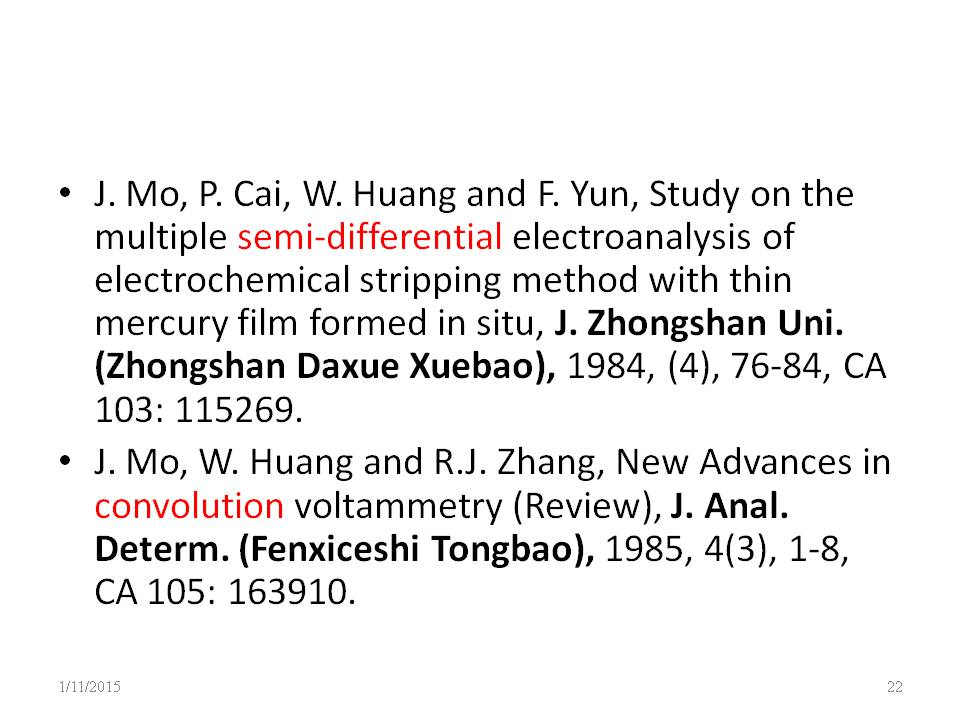Two times of semiderivative equal to first derivative,
d^0.5/dx^0.5 d^0.5/dx^0.5 sin(x) = d/dx sin(x) = cos(x)

3. ## Complex order derivative

The order can be extented to complex number, the (1+i) order derivative and the (1-i) order derivative equal to second derivative,
d^(1+i)/dx^(1+i) d^(1-i)/dx^(1-i) sin(x) = d^2/dx^2 sin(x) = -sin(x)
4. ## Difference between Caputo definition and the Riemann-Liouville (R-L) definition

The Caputo (Davison-Essex) and the Riemann-Liouville definitions are different in the following aspect: in the Caputo formula, differentiation is performed first, then integration; but in the R-L formula it is the other way around. The Caputo definition has advantages over the R-L definition:
1. The Caputo definition implemented maps constants to zero, imitating integer order differentiation, while the R-L definition does not.
2. The Caputo definition requires initial value in fractional differential equation is the same as in differential equations, while the R-L definition does not.
These properties of the Caputo definition make it suitable to work with initial value problems for fractional differential equations. So the Caputo definition is used here. If you want to use the Riemann defintion, use the Laplace transform solver lasolve().
lasolve(y(-0.5,x)=1) give nonzero.

6. ## Application

It has been used in many areas, e.g. in electrochemistry, see above last picture.
﻿